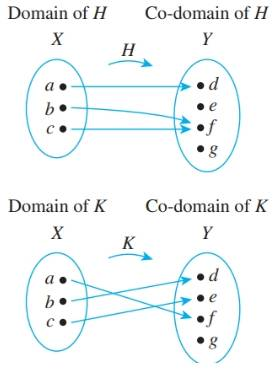Chapter 7.2, Problem 8ES### Discrete Mathematics With Applicat...

5th Edition
EPP + 1 other
ISBN: 9781337694193

#### Solutions

Chapter
Section### Discrete Mathematics With Applicat...

5th Edition
EPP + 1 other
ISBN: 9781337694193
Textbook Problem
1 views

# Let X = { a ,   b ,   c } and Y = { d ,   e ,   f ,   g } . Define functions H and K by the arrow diagrams belowa. Is H one-to-one? Why or why not? Is it onto? Why or why not? b. Is K one-to-one? Why or why not? Is it onto? Why or why not?

To determine

(a)

To check:

Whether H is one-to-one or onto.

Explanation

Given information:

The arrow diagram of given function H is as follows:

Concept used:

A function F is said to be one-to-one function if and only if, the distinct elements in its domain are mapped with the distinct elements in its co-domain.

A function is said to be onto function if and only if, each element in its codomain is the image of at least one element in its domain.

Calculation:

The domain of the function H is X={a,b,c} and co domain is Y={d,e,f,g}.

The function H is defined as H(a)=d,H(b)=f,H(c)=f.

The objective is to find if the function H is one to one.

One to one function: A function F:XY is said to be one to one if and only if, x1,x2X

If F(x1)=F(x2), then x1=x2.

For the function H ,

H(b)=H(c)=y

But bc

To determine

(b)

Whether the function K is one-to-one or not and explain the reason for the same. Also, if the function is onto or not and explain the reason for the same.

### Still sussing out bartleby?

Check out a sample textbook solution.

See a sample solution

#### The Solution to Your Study Problems

Bartleby provides explanations to thousands of textbook problems written by our experts, many with advanced degrees!

Get Started